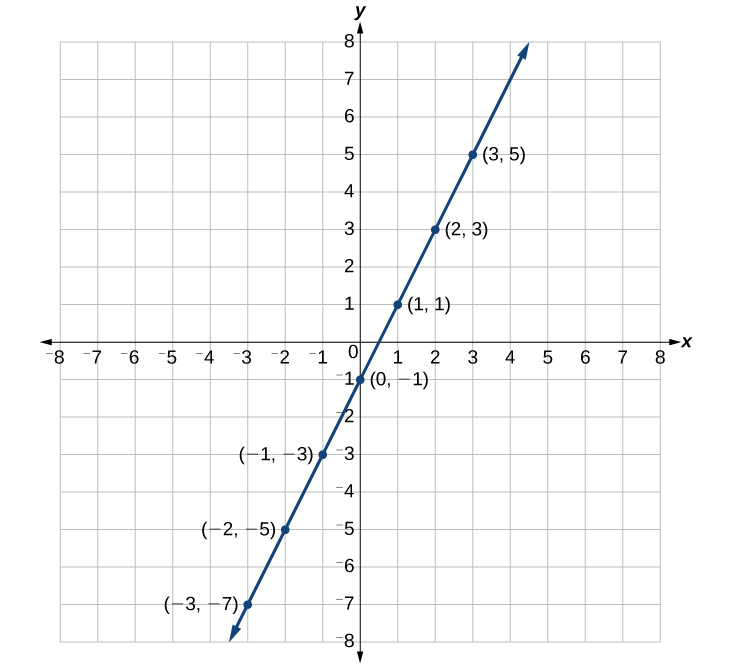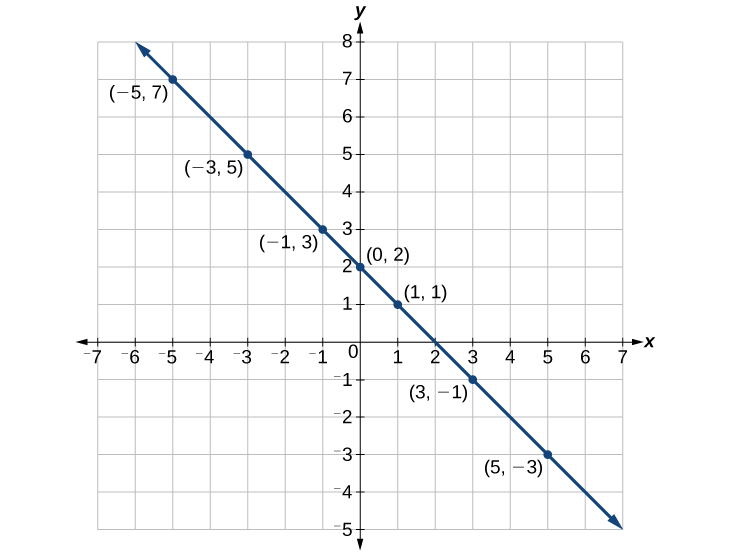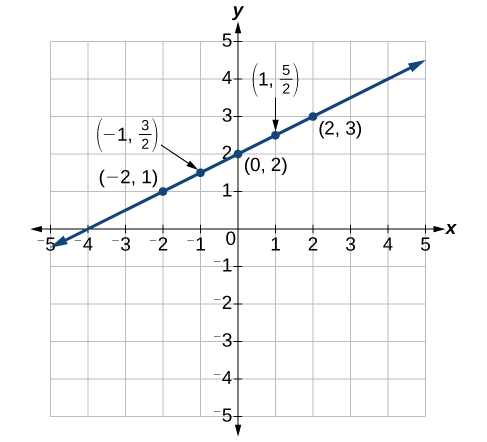## Graphing Equations by Plotting Points

We can plot a set of points to represent an equation. When such an equation contains both an x variable and a y variable, it is called an equation in two variables. Its graph is called a graph in two variables. Any graph on a two-dimensional plane is a graph in two variables.

Suppose we want to graph the equation $y=2x - 1$. We can begin by substituting a value for x into the equation and determining the resulting value of y. Each pair of x– and y-values is an ordered pair that can be plotted. The table below lists values of x from –3 to 3 and the resulting values for y.

 $x$ $y=2x - 1$ $\left(x,y\right)$ $-3$ $y=2\left(-3\right)-1=-7$ $\left(-3,-7\right)$ $-2$ $y=2\left(-2\right)-1=-5$ $\left(-2,-5\right)$ $-1$ $y=2\left(-1\right)-1=-3$ $\left(-1,-3\right)$ $0$ $y=2\left(0\right)-1=-1$ $\left(0,-1\right)$ $1$ $y=2\left(1\right)-1=1$ $\left(1,1\right)$ $2$ $y=2\left(2\right)-1=3$ $\left(2,3\right)$ $3$ $y=2\left(3\right)-1=5$ $\left(3,5\right)$

We can plot the points in the table. The points for this particular equation form a line, so we can connect them. This is not true for all equations.Figure 6

Note that the x-values chosen are arbitrary, regardless of the type of equation we are graphing. Of course, some situations may require particular values of x to be plotted in order to see a particular result. Otherwise, it is logical to choose values that can be calculated easily, and it is always a good idea to choose values that are both negative and positive. There is no rule dictating how many points to plot, although we need at least two to graph a line. Keep in mind, however, that the more points we plot, the more accurately we can sketch the graph.

### How To: Given an equation, graph by plotting points.

1. Make a table with one column labeled x, a second column labeled with the equation, and a third column listing the resulting ordered pairs.
2. Enter x-values down the first column using positive and negative values. Selecting the x-values in numerical order will make the graphing simpler.
3. Select x-values that will yield y-values with little effort, preferably ones that can be calculated mentally.
4. Plot the ordered pairs.
5. Connect the points if they form a line.

### Example 2: Graphing an Equation in Two Variables by Plotting Points

Graph the equation $y=-x+2$ by plotting points.

### Solution

First, we construct a table similar to the one below. Choose x values and calculate y.

 $x$ $y=-x+2$ $\left(x,y\right)$ $-5$ $y=-\left(-5\right)+2=7$ $\left(-5,7\right)$ $-3$ $y=-\left(-3\right)+2=5$ $\left(-3,5\right)$ $-1$ $y=-\left(-1\right)+2=3$ $\left(-1,3\right)$ $0$ $y=-\left(0\right)+2=2$ $\left(0,2\right)$ $1$ $y=-\left(1\right)+2=1$ $\left(1,1\right)$ $3$ $y=-\left(3\right)+2=-1$ $\left(3,-1\right)$ $5$ $y=-\left(5\right)+2=-3$ $\left(5,-3\right)$

Now, plot the points. Connect them if they form a line.Figure 7

Construct a table and graph the equation by plotting points: $y=\frac{1}{2}x+2$.

 $x$ $y=\frac{1}{2}x+2$ $\left(x,y\right)$ $-2$ $y=\frac{1}{2}\left(-2\right)+2=1$ $\left(-2,1\right)$ $-1$ $y=\frac{1}{2}\left(-1\right)+2=\frac{3}{2}$ $\left(-1,\frac{3}{2}\right)$ $0$ $y=\frac{1}{2}\left(0\right)+2=2$ $\left(0,2\right)$ $1$ $y=\frac{1}{2}\left(1\right)+2=\frac{5}{2}$ $\left(1,\frac{5}{2}\right)$ $2$ $y=\frac{1}{2}\left(2\right)+2=3$ $\left(2,3\right)$Figure 8